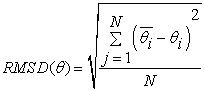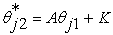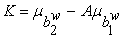﻿ Accuracy of Parameter Estimation and Concordance Method Based on Item Response Theory

### Accuracy of Parameter Estimation and Concordance Method Based on Item Response Theory

Wardani RahayuOPEN ACCESSPEER-REVIEWED

## Accuracy of Parameter Estimation and Concordance Method Based on Item Response Theory

Wardani RahayuMathematics and Science Department, State University of Jakarta, Indonesia, Kampus UNJ, Jl. Rawamangun Muka, Rawamangun, Jakarta

### Abstract

The objective of this study was to investigate estimation and concordance method’s accuracy parameter based on item response theory. Estimation method used were Joint Maximum Likelihood, Bayesian and Bayesian Modal; and concordance method used were mean and sigma method, and robust mean and sigma method with sample size of 500 and 800. Data source used was test of tryout of Senior High School year 2012. Hypothesis testing on comparative values of root mean square difference (RMSD) using one way anova and t test. The results were parameter estimation method of Joint Maximum Likelihood, Bayesian and Bayes Modal on two logistic model parameters that produced similar estimation result using sample size more than 500, robust mean and sigma method was more accurate than mean and sigma method.

### At a glance: Figures

1
Prev Next

• Rahayu, Wardani. "Accuracy of Parameter Estimation and Concordance Method Based on Item Response Theory." American Journal of Educational Research 3.5 (2015): 552-555.
• Rahayu, W. (2015). Accuracy of Parameter Estimation and Concordance Method Based on Item Response Theory. American Journal of Educational Research, 3(5), 552-555.
• Rahayu, Wardani. "Accuracy of Parameter Estimation and Concordance Method Based on Item Response Theory." American Journal of Educational Research 3, no. 5 (2015): 552-555.

 Import into BibTeX Import into EndNote Import into RefMan Import into RefWorks

### 1. Introduction

One of the mathematics nature is both a king and servant of science. It has the meaning as a tool and servant of other sciences. The fact, concept, principle and procedure of mathematics are commonly used as a support to the other science field’s concept and principle development such as natural science, technical science, medical science, and social science such as economics and psychology. Therefore mathematics is always included as a course or a compulsory since Early Childhood Education (ECE), Elementary School, up to Universities.

The numbers of hours provided by school has not given any satisfactory result. Students have not been able to apply the concept, fact, principle and procedure of mathematics at school in order to do problem solving in daily life. The students are only able to solve low category mathematic question which is in the cognitive area of recollecting, understanding, and application, while the students are not use to solve the questions in higher order thinking category, which is in the cognitive area of analysis, synthesis, evaluation and creativity. It can be seen from numbers of international surveys regarding with the students ability in Indonesia such as Trends in International Math and Science’ Global Institute survey’s result in 2007, which states that there are 5 percent of students in Indonesia who are able to solve questions which need a high level of reasoning and there are 78 percent of students in Indonesia who are able to solve questions in the cognitive area of recollecting. It is different from the students in Korea where 71 percent of them can solve questions in higher order thinking category.  This result is also supported by the study of Frederick from The University of Hongkong stating that the majority of questions given by the mathematics teacher in Indonesia are too rigid. Generally, students in Indonesia are given some questions which are expressed in language and mathematics symbol set in the context which is far from the reality of daily life. 

Teacher Education Consensus Point of mathematics and other private institutions have developed mathematics question including cognitive area of recollecting, understanding, application and analysis for Senior High School try out test based on the passing grade standard which has been developed by National Education Standards Board. Each Teacher Education Consensus Point of mathematics regional and private institutions develop their own outline content so that the developed indicator will be different. These mathematics test results cannot be directly compared between one from the regency and one from the city, due to the different measurement contruction. Therefore, the score equating which is known as concordance is needed to be performed. This score linking is used to relate the scores on test which is constructed in a different construction. [3, 4] Hence, the result score of concordance which considered appropriate may not be interpreted as equating result. Equating is performed on two tests which are measuring the same construction [5, 6], although these two test are having a different difficulty level on each items which are measuring the same construction.  The same linking method can be used in concordance through two approaches namely classic test theory and item response theory.

Retnawati (2012) compares three methods of concordance by using classical theory approach, namely linear method, parallel linear method, and equipercentile method.  Candell and Drasgown (1988), Cohen and Kim (1992), Rahayu (2010), compare the linking method related with DIF detection based on item response theory [8, 9, 10].

Score linking on item response theory may use mean and sigma, robust mean and sigma, characteristics curve  and chi square minimum method.  Score linking by using concordance method includes the estimation result of item difficulty parameter, item discrimination and the ability of test participants. Item parameter estimation can be pursued by three methods namely Joint Maximum Likelihood method, Bayesian method and Bayes Modal method. The problem arising is if the item parameter is done with two different methods, namely concordance and parameter estimation method, then which method is the most accurate?

### 2. Method

The study is in form of experimental research which consists of three independent variables, namely concordance method, parameter estimation method and sample size. The dependent variable from this study is the RMSD score. The concordance method which is used in this research is mean and sigma method, and robust mean and sigma method. parameter Estimation methods are Joint maximum Likelihood, Bayesian and Bayes Modal method. The logistics method which is used is one parameter logistics model (L2P) with the sample size of 500 and 800.

The data used in this research is the try out score year 2012 from North Jakarta and South Jakarta areas. This students work’s result score is in form of 40 multiple choices of A, B, C, D, and E questions. The responses of try out participants are originate from each area that were done on 1000 responses replication.

According to Kolen and Brennan (1995), the failure of scale equating on concordance is declared as the difference between the results of the real concordance compared with the expectation score.  The accuracy of concordance method can be seen from the average value of root mean square difference (RMSD), the smaller RMSD average value on mean and sigma method, and robust mean and sigma method which is summarized that one of such concordance methods is the most accurate. RSMD is determined with the following formula[14, 15]

N = size of sample.= the ability of participant i after the equating.= the ability of participant i before the equating.

Mean and sigma method using transformation equation areRobust mean and sigma method transformation equation are### 3. Results and Discussion

From concordance with the mean and sigma method by using 500 sample size, RSMD distribution (Figure 1) shows that the Joint Maximum Likelihood, Bayesian and Bayes Modal method, and almost all RSMD scores are under the median score. It can be interpreted that these three estimation parameter methods are having the same accuracy. The different is found in 800 sample size; the RSMD on Bayesian method has more homogeny distribution compared with Joint Maximum Likelihood and Bayes Modal method. Therefore, it can be said descriptively that Bayesian method is more accurate compares to Joint Maximum Likelihood and Bayes Modal method.

From the concordance with robust mean and sigma method by using 500 sample size, RSMD distribution (Figure 1) shows that Bayesian and Bayes modal method is almost similar and most of the RSMD scores are on the low score below the median score. It can be interpreted that these Bayesian and Bayes Modal method are having almost the same accuracy. Whereas, descriptively, the Bayesian and Bayes modal method are more accurate if compared with Joint Maximum Likelihood method. On 800 sample size, based on Figure 2, it can be summarized that these Bayesian and Bayes Modal method are having almost the same accuracy and both more accurate compared to Joint Maximum Likelihood method.

Concordance with mean and sigma method, robust mean and sigma method with Joint Maximum Likelihood, Bayesian or Bayes modal method, and the 800 sample size RMSD distribution is more homogeny compared with 500 sample size. It can be summarized that the bigger the sample size, the more accurate concordance and parameter estimation method.

On the concordance with mean and sigma method by using 500 and 800 sample size, it can be seen on table 1 that the sign value > 0.05, therefore the RSMD score for Joint Maximum Likelihood, Bayesian and Bayes Modal method is not different. It can be interpreted that the three parameter estimation methods have the same accuracy on more than 500 samples by using this mean and sigma method.

#### Table 2. Test Result of One Way Anova On Concordance

The concordance with mean and sigma method, and robust mean and sigma by using 500 and 800 sample size refers to sign scores (Table 2). Therefore, the RSMD score for Joint Maximum Likelihood, Bayesian and Bayes Modal method are not different. It can be interpreted that the three estimation parameter methods by using mean and sigma method, and robust mean and sigma method are having the same accuracy for more than 500 samples.

On Joint Maximum Likelihood method by using 500 and 800 sample size, the sign score is less than 0.05 and the average score of RSMD of mean and sigma method is more than the average score of RSMD of robust mean and sigma method. Therefore, it can be interpreted that the robust mean and sigma method is more accurate than mean and sigma method.

On Bayes Modal method by using 500 and 800 sample size, the sign score is less than 0.05 and the average score of RSMD of mean and sigma method is more than the average score of RSMD of robust mean and sigma method. Therefore, it can be interpreted that the robust mean and sigma method is more accurate than mean and sigma method. Therefore, it can be summarized that robust mean and sigma method is more accurate than mean and sigma method by using estimation parameter method of Joint Maximum Likelihood, Bayesian and Bayes Modal method on item response theory on more than 500 sample size.

#### Table 3. Results of t test On Estimation Parameter Method

The Joint Maximum Likelihood method, Bayesian method and Bayes Modal method are parameter estimation methods which are applied in this research; mean and sigma method, and robust mean and sigma method for the concordance shows the accuracy of estimation parameter method and concordance by using RSME. The less RSMD, the more accurate the parameter estimation and concordance methods.

The result of this research shows that between the three estimation parameter methods, this robust mean and sigma method and mean and signa method have the same accuracy level on more than 500 sample size. Therefore, the Joint Maximum Likelihood, Bayesian or Bayes Modal can be used to estimates the parameter because they are having almost similar estimation parameter results.

The next result of this research shows that concordance by using robust mean and sigma method is more accurate than using mean and sigma method on more than 500 sample size. On robust mean and sigma method,is a coefficient that obtained from the comparison between standard deviation of scored item difficulty from the two groups,is a coefficient that obtained from scored item difficulty’s mean difference of the second group and A multiplication with the first group’s scored item difficulty. First and second group’s weighted item difficulty in item to-j was obtained from the difficulty multiplication with the score scales. Thus the score scale to-j has a value between 0 and 1 so that scored item difficulty less than non-scored item difficulty value. While the concordance results with mean and sigma method using non-weighted item difficulty and item difficulty estimation’s result with different accuracy degree .

Therefore, robust mean and sigma method can be used in order to perform the score linking from two different tests with two different construct measurements by using the Joint Maximum Likelihood and Bayesian or Bayes Modal methods.

### 4. Conclusion

Parameter estimation methods, such as Joint Maximum Likelihood, Bayesian and Bayes Modal methods on two parameter’s logistics model parameters result in almost similar estimation scores by using more than 500 sample size. On concordance, robust mean and sigma method is more accurate than mean and sigma method.

### References

  http://fokus.news.viva.co.id/news/read/371744-kurikulum-pendidikan-2013--apa-yang-baru- (accessed January 1, 2013).In article  http://digilib.unimed.ac.id/public/UNIMED-Master-28731-081188710008%20Bab%20I.pdf, p. 5-6. (accessed January 1, 2013).In article  Pommerich, Mary; Bradley A. Hanson, Deborah J. Harris and James A. Sconing, “Issues in Conducting Linkages Between Distinct Tests.” Applied Psychological Measurement, 28 (4), 247-273. 2004.In article CrossRef  Kolen, Michael J and Robert L Brenan, Test Equating, Scaling and Linking. Springer, New York, 2004.In article  Von Davier, Alina A, Statistical for Test Equating, Scaling, and Linking, Springer, New York, 2011.In article CrossRef  Dorrans, Neil J., “Equating, Concordance, and Expectation,” Applied Psychological Measurement, 28 (4), 227-246. 2004.In article CrossRef  Retnawati, Heri and Kana Hidayati, Perbandingan Metode Concordance Berdasarkan Teori Tes Klasik, http://staff.uny.ac.id/sites/ (accessed January 1, 2013).In article  Candell, Gregory L. and Frits Drasgow, “An Iterative Procedure for Linking Metrics and Assensing Item Bias in Item Response Theory,” Applied Psychological Measurement, 12 (3), 253-260, 1988.In article CrossRef  Cohen, Allan S. and Seock-Ho Kim, “Effect of Linking Methods on Detection of DIF,” Journal of Educational Measurement, 29 Issue 1, 51-66. 1992.In article  Rahayu, Wardani, “Metode Linking dan Butir False Positive Pada Pendekteksian DIF Berdasarkan Teori Responsi Butir,” Jurnal Penelitian dan Evaluasi Pendidikan, Nomor 1 Tahun 14, 21-36. 2010.In article  Hambleton, Ronald K. dan H Swaminathan, Item Response Theory: Principles and Aplications, Kluwer, Boston, 1995.In article  Divgi, D.R., “Minimum Chi-Square Method for Developing a Common Metric in Item Response Theory,’ Journal Applied Psychological Measurement, 9(4), 413-415. 1985.In article CrossRef  Kolen, Michael and Robert L Brenan, Test Equating, Springer, New York, 1995.In article  Kartono, “Equating The Combined Dichotomous And Politomous Item Test Model In An Achievement Test,” Jurnal Penelitian dan Evaluasi Pendidikan, Nomor 2 Tahun XII, 302-320. 2008.In article  Cohen, Allan S. and Seock-Ho Kim, “Comparison of Linking and Concurenrent Calibration Under Item Rersponse Theory,” Applied Psychological Measurement, 26(1), 131-143. 2002.In article  Kim, Jee-Seon Kim and Bradley A. Hanson, “Test Equating the Multiple-Choice Model’, Applied Psychological Measurement, 26 (3), 255-270. 2002.In article CrossRef  Hambleton, R. K., H. Swaminathan dan Jane Rogers, Fundamentals of Item Response Theory, Sage, Newbury Park, CA, 1991.In article